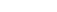# Bayesian Analysis

•An Introduction to Lifted Probabilistic Inference \$70.00
•Bayesian Statistics for Experimental Scientists (A General Introduction Using Distribution-Free Methods) \$65.00
•Bayesian Statistics the Fun Way (Understanding Statistics and Probability with Star Wars, LEGO, and Rubber Ducks) \$34.95
•Chancing It (The Laws of Chance and How They Can Work for You) \$24.99
•Doing Data Science (Straight Talk from the Frontline) \$39.99
•The Doomsday Calculation (How an Equation that Predicts the Future Is Transforming Everything We Know About Life and the Universe) \$29.00
•The Doomsday Calculation (How an Equation that Predicts the Future Is Transforming Everything We Know About Life and the Universe) - 9780316440691 \$18.99
•The Subjectivity of Scientists and the Bayesian Approach \$19.95
•Think Bayes (Bayesian Statistics in Python) \$29.99
•Think Bayes (Bayesian Statistics in Python) - 9781492089469 \$49.99